## Homework 4

1. The 1D model of a crystal puts the following constraint on the wave number.Assume thatand plot the constraint as a function of. Plot the allowed energy bands on an energy axis assumingeV and the spacing between atoms is 5 Angstroms.

2. In a 1D square well, there is always at least one bound state. Assume the width of the square well is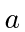. By the uncertainty principle, the kinetic energy of an electron localized to that width is. How can there be a bound state even for small values of?

3. Ata particle is in the one dimensional Harmonic Oscillator state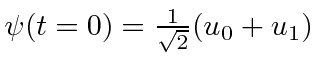. Iscorrectly normalized? Compute the expected value ofas a function of time by doing the integrals in therepresentation.

4. Prove the Schwartz inequality. (Start from the fact thatfor any.

5. The hyper-parity operatorhas the property that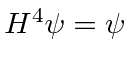for any state. Find the eigenvalues offor the case that it is not Hermitian and the case that it is Hermitian.

6. Find the correctly normalized energy eigenfunction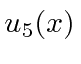for the 1D harmonic oscillator.

7. A beam of particles of energycoming from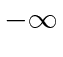is incident upon a double delta function potential in one dimension. That is.
a)
Find the solution to the Schrödinger equation for this problem.
b)
Determine the coefficients needed to satisfy the boundary conditions.
c)
Calculate the probability for a particle in the beam to be reflected by the potential and the probability to be transmitted.

Jim Branson 2013-04-22